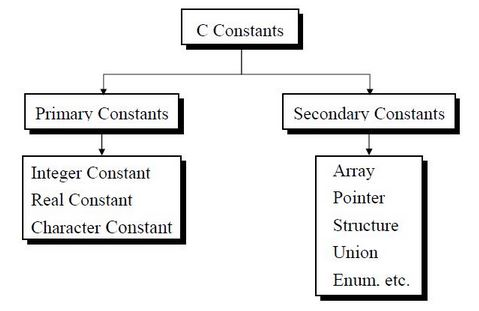The C Constants

In C constants are of two types.
1. Primary constant.
2. Secondary constant.Rules for integer constant:

• An integer constant must have at least one digit.
• It must not have a decimal point.
• It can be either positive or negative.
• If no sign precedes an integer constant it is assumed to be positive.
• No commas or blanks are allowed within an integer constant.
• The allowable range for integer constants is -32768 to 32767.

The range vary from compiler to compiler. Here the range is given for 16 bit compiler.

Rules for real constant:

• A real constant must have at least one digit.
• It must have a decimal point.
• It could be either positive or negative.
• Default sign is positive.
• No commas or blanks are allowed within a real constant.
• The range of real constant is -3.4e38 to 3.4e38. (Where part before e is called mantissa   and part after e is called exponent)

• Rules for character constant:

• Character constant is a single alphabet, a single digit or a single special symbol enclosed within single inverted commas. Both the inverted commas should point to the left. For example, ’A’ is a valid character constant whereas ‘A’ is not.
• The maximum length of a character constant can be 1 character.

The range vary from compiler to compiler. Here the range is given for 16 bit compiler.

C Example

#include<stdio.h>

int main() {
int i=1;
float a=2.1;
char c='A';
printf("%d",i);
return 0;
}